# Exercises: Chapter 2, Section 6

1. Use the implicit function theorem to re-do Problem 2-15(c).

Define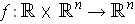by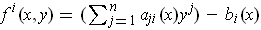for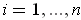. One has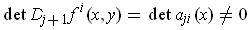for all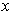. The determinant condition guarantees that for each, there is exactly one solution of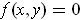, call that solution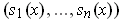.

Now, for each, the Implicit function theorem says that there is a function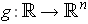defined in an open neighborhood ofand such that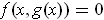and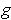is differentiable. By the uniqueness of the solutions in the last paragraph, it must be that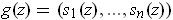for all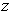in the domain of. In particular, the various functionsall glue together into a single function defined on all of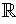and differentiable everywhere.

By differentiating the relation, one gets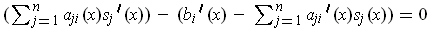for. Note that this is of the same form as the set of equations for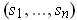except that the right hand side functions have changed. An explicit formula can be obtained by using Cramer's rule.

2. Let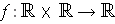be differentiable. For each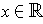defined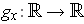by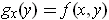. Suppose that for eachthere is a unique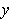with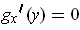; let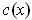be this.

In this problem, it is assumed that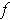was meant to be continuously differentiable.

1. If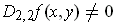for all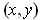, show that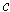is differentiable and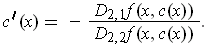Just as in the last problem, the uniqueness condition guarantees thatis the same as the function provided by the implicit function theorem applied to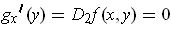. In particular,is differentiable and differentiating this last relation gives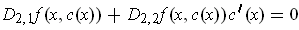. Solving for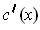gives the result.

2. Show that if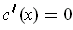, then for somewe have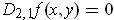and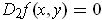.

This follows immediately from part (a).

3. Let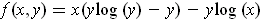. Find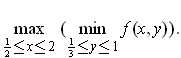Note that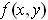is defined only whenandare positive. So we need to go back and show that the earlier parts of the problem generalize to this case; there are no difficulties in doing this.

One has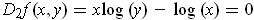precisely when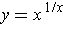and so the hypothesis of part (a) is true and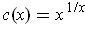. Also,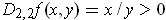(since bothandare positive), and so for fixed, the minimum ofoccurs atby the second derivative test. Now actually, we are not looking for the minimum over all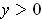, but just for those in the interval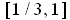. The derivative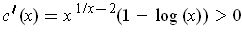for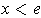. Further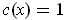precisely when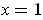and there is a unique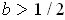where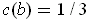. For fixed,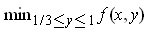is achieved at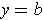if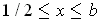, at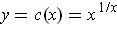if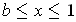, and at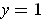if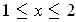.

We will find where the maximum of the minimum's are located in each of the three cases.

Suppose. Then we need to maximize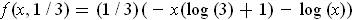. The derivative of this function is negative throughout the interval; so the maximum occurs at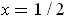. The maximum value is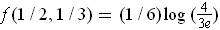.

Suppose. Then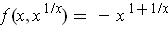. The derivative of this function is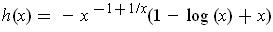. This function has no zeros in the interval because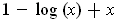has derivative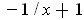which is always negative in the interval and the value of the function is positive at the right end point. Now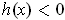on the interval, and so the maximum must occur at the left hand end point. The maximum value is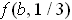. In view of the last paragraph, that means that the maximum over the entire interval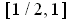occurs at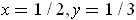.

Suppose. Then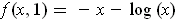. This is a decreasing function and so the maximum occurs at the left hand endpoint. By the result of the previous paragraph the maximum over the entire interval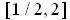must therefore occur at,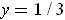, and the value of the maximum is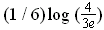.